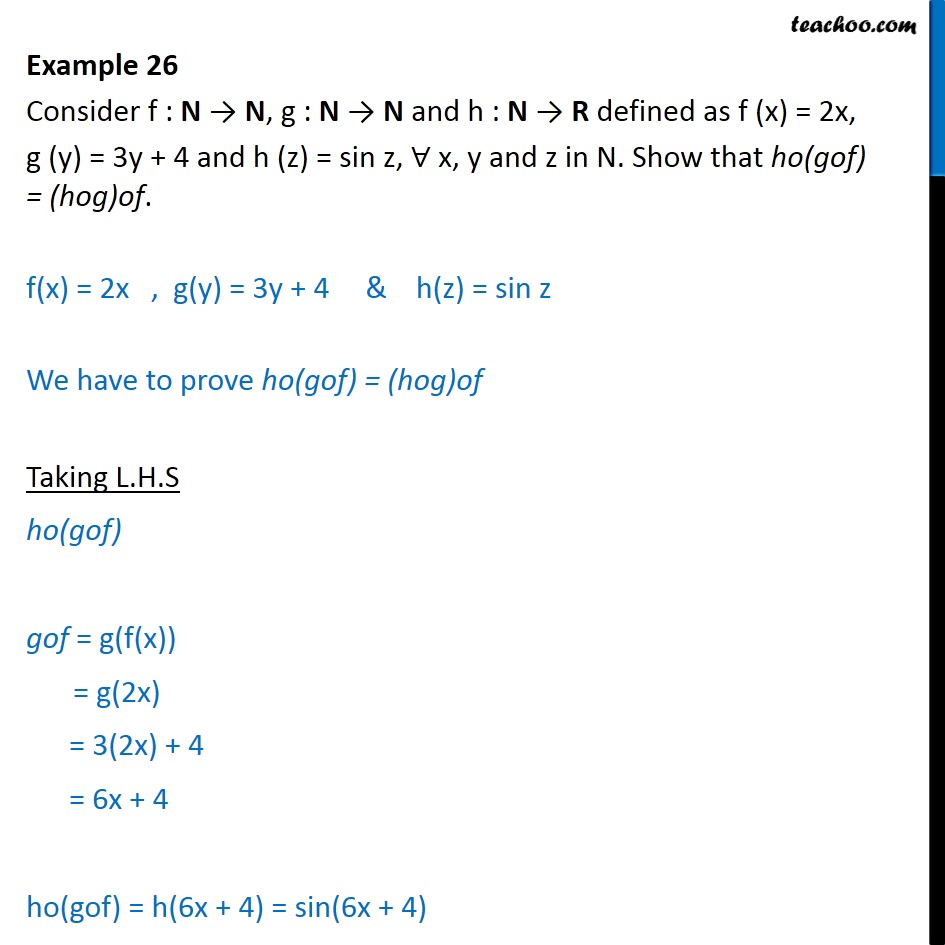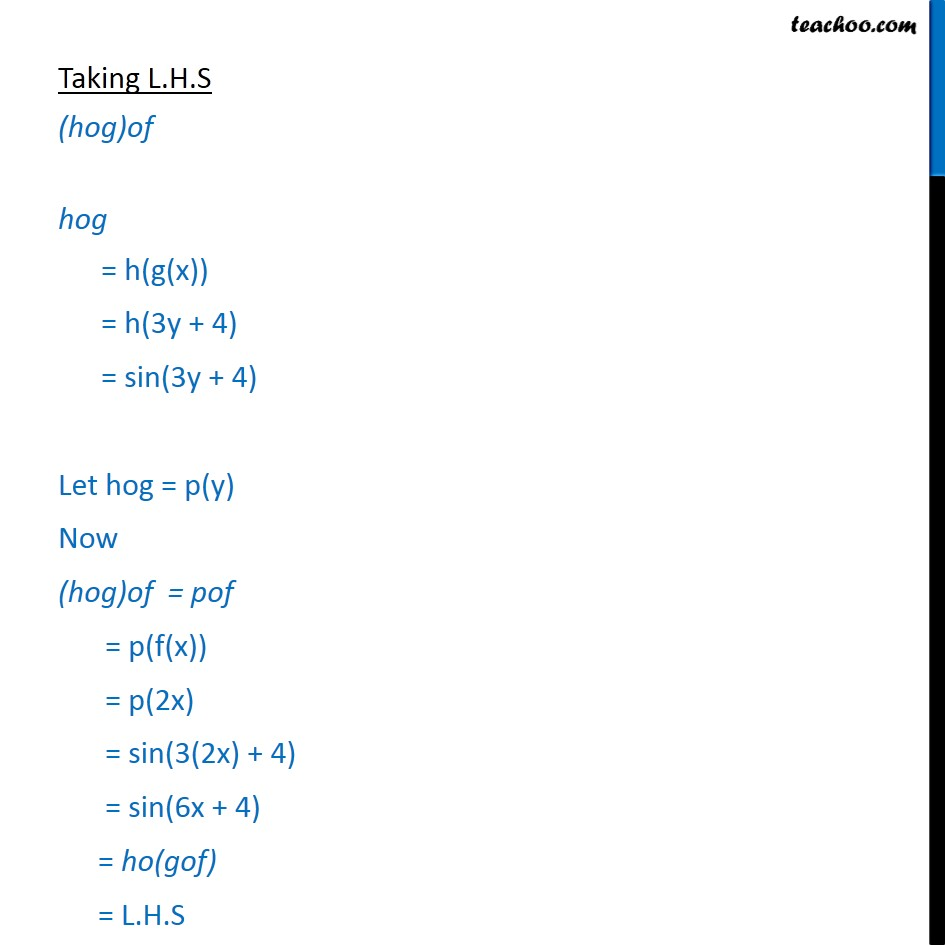Composite functions

Chapter 1 Class 12 Relation and Functions
Concept wiseIntroducing your new favourite teacher - Teachoo Black, at only ₹83 per month

### Transcript

Example 26 Consider f : N → N, g : N → N and h : N → R defined as f (x) = 2x, g (y) = 3y + 4 and h (z) = sin z, ∀ x, y and z in N. Show that ho(gof) = (hog)of. f(x) = 2x , g(y) = 3y + 4 & h(z) = sin z We have to prove ho(gof) = (hog)of Taking L.H.S ho(gof) gof = g(f(x)) = g(2x) = 3(2x) + 4 = 6x + 4 ho(gof) = h(6x + 4) = sin(6x + 4) Taking L.H.S (hog)of hog = h(g(x)) = h(3y + 4) = sin(3y + 4) Let hog = p(y) Now (hog)of = pof = p(f(x)) = p(2x) = sin(3(2x) + 4) = sin(6x + 4) = ho(gof) = L.H.S Since L.H.S = R.H.S Hence proved